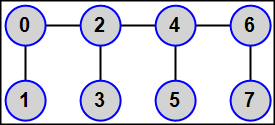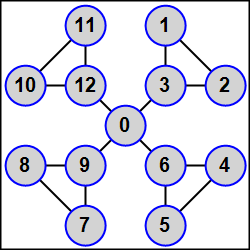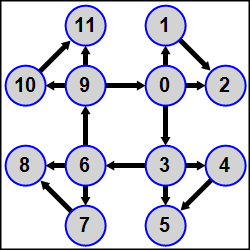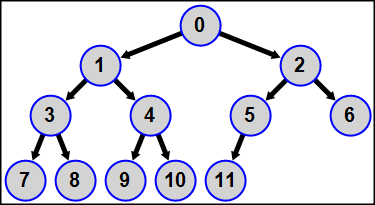Russian1100 training tasks on programming

©  M. E. Abramyan (Southern Federal University), 1998–2022

 Main Tasks Examples PT for MPI-2

 Overview Task groups MPI1Proc MPI2Send MPI3Coll MPI4Type MPI5Comm MPI6File MPI7Win MPI8Inter MPI9Matr MPIDebug Solution example Basic concepts Template creation Program running Task solution Using debug section
 PT for MPI-2 | Task groups | MPI5Comm

# Process groups and communicators

## Creation of new communicators

MPI5Comm1°. A sequence of K integers is given in then master process; K is the number of processes whose rank is an even number (0, 2, …). Create a new communicator that contains all even-rank processes using the MPI_Comm_group, MPI_Group_incl, and MPI_Comm_create functions. Send one given integer to each even-rank process (including the master process) using one collective operation with the created communicator. Output received integer in each even-rank process.

MPI5Comm2°. Two real numbers are given in each process whose rank is an odd number (1, 3, …). Create a new communicator that contains all odd-rank processes using the MPI_Comm_group, MPI_Group_excl, and MPI_Comm_create functions. Send all given numbers to each odd-rank process using one collective operation with the created communicator. Output received numbers in each odd-rank process in ascending order of ranks of sending processes (including numbers received from itself).

MPI5Comm3°. Three integers are given in each process whose rank is a multiple of 3 (including the master process). Using the MPI_Comm_split function, create a new communicator that contains all processes with ranks that are a multiple of 3. Send all given numbers to master process using one collective operation with the created communicator. Output received integers in the master process in ascending order of ranks of sending processes (including integers received from the master process).

Note. When calling the MPI_Comm_split function in processes that are not required to include in the new communicator, one should specify the constant MPI_UNDEFINED as the color parameter.

MPI5Comm4°. A sequence of 3 real numbers is given in each process whose rank is an even number (including the master process). Find the minimal value among the elements of the given sequences with the same order number using a new communicator and one global reduction operation. Output received minimums in the master process.

Note. See the note to MPI5Comm3.

MPI5Comm5°. A real number is given in each process. Using the MPI_Comm_split function and one global reduction operation, find the maximal value among the numbers given in the even-rank processes (including the master process) and the minimal value among the numbers given in the odd-rank processes. Output the maximal value in the process 0 and the minimal value in the process 1.

Note. The program should contain a single MPI_Comm_split call, which creates the both required communicators (each for the corresponding group of processes).

MPI5Comm6°. An integer K and a sequence of K real numbers are given in the master process, an integer N is given in each slave process. The value of N is equal to 1 for some processes and is equal to 0 for others; the number of processes with N = 1 is equal to K. Send one real number from the master process to each slave process with N = 1 using the MPI_Comm_split function and one collective operation. Output the received numbers in these slave processes.

Note. See the note to MPI5Comm3.

MPI5Comm7°. An integer N is given in each process; the value of N is equal to 1 for at least one process and is equal to 0 for others. Also a real number A is given in each process with N = 1. Send all numbers A to the first process with N = 1 using the MPI_Comm_split function and one collective operation. Output received numbers in this process in ascending order of ranks of sending processes (including the number received from this process).

Note. See the note to MPI5Comm3.

MPI5Comm8°. An integer N is given in each process; the value of N is equal to 1 for at least one process and is equal to 0 for others. Also a real number A is given in each process with N = 1. Send all numbers A to the last process with N = 1 using the MPI_Comm_split function and one collective operation. Output received numbers in this process in ascending order of ranks of sending processes (including the number received from this process).

Note. See the note to MPI5Comm3.

MPI5Comm9°. An integer N is given in each process; the value of N is equal to 1 for at least one process and is equal to 0 for others. Also a real number A is given in each process with N = 1. Send all numbers A to each process with N = 1 using the MPI_Comm_split function and one collective operation. Output received numbers in these processes in ascending order of ranks of sending processes (including the number received from itself).

Note. See the note to MPI5Comm3.

MPI5Comm10°. An integer N is given in each process; the value of N is equal to 1 for some processes and is equal to 2 for others, there are at least one process with N = 1 and one process with N = 2. Also an integer A is given in each process. Using the MPI_Comm_split function and one collective operation, send integers A from all processes with N = 1 to each process with N = 1 and from all processes with N = 2 to each process with N = 2. Output received integers in each process in ascending order of ranks of sending processes (including the integer received from itself).

Note. See the note to MPI5Comm5.

MPI5Comm11°. An integer N is given in each process; the value of N is equal to 1 for at least one process and is equal to 0 for others. Also a real number A is given in each process with N = 1. Find the sum of all real numbers A using the MPI_Comm_split function and one global reduction operation. Output the received sum in each process with N = 1.

Note. See the note to MPI5Comm3.

MPI5Comm12°. An integer N is given in each process; the value of N is equal to 1 for some processes and is equal to 2 for others, there are at least one process with N = 1 and one process with N = 2. Also a real number A is given in each process. Using the MPI_Comm_split function and one global reduction operation, find the minimal value among the numbers A given in the processes with N = 1 and the maximal value among the numbers A given in the processes with N = 2. Output the minimal value in each process with N = 1 and the maximal value in each process with N = 2.

Note. See the note to MPI5Comm5.

## Virtual topologies

MPI5Comm13°. An integer N (> 1) is given in the master process; the number of processes K is assumed to be a multiple of N. Send the integer N to all processes and define a Cartesian topology for all processes as a (N × K/N) grid using the MPI_Cart_create function (ranks of processes should not be reordered). Find the process coordinates in the created topology using the MPI_Cart_coords function and output the process coordinates in each process.

MPI5Comm14°. An integer N (> 1) is given in the master process; the number N is not greater than the number of processes K. Send the integer N to all processes and define a Cartesian topology for the initial part of processes as a (N × K/N) grid using the MPI_Cart_create function (the symbol "/" denotes the operator of integer division, ranks of processes should not be reordered). Output the process coordinates in each process included in the Cartesian topology.

MPI5Comm15°. The number of processes K is an even number: K = 2N, N > 1. A real number A is given in the processes 0 and N. Define a Cartesian topology for all processes as a (2 × N) grid. Using the MPI_Cart_sub function, split this grid into two one-dimensional subgrids (namely, rows) such that the processes 0 and N were the master processes in these rows. Send the given number A from the master process of each row to each process of the same row using one collective operation. Output the received number in each process (including the processes 0 and N).

MPI5Comm16°. The number of processes K is an even number: K = 2N, N > 1. A real number A is given in the processes 0 and 1. Define a Cartesian topology for all processes as a (N × 2) grid. Using the MPI_Cart_sub function, split this grid into two one-dimensional subgrids (namely, columns) such that the processes 0 and 1 were the master processes in these columns. Send the given number A from the master process of each column to each process of the same column using one collective operation. Output the received number in each process (including the processes 0 and 1).

MPI5Comm17°. The number of processes K is a multiple of 3: K = 3N, N > 1. A sequence of N integers is given in the processes 0, N, and 2N. Define a Cartesian topology for all processes as a (3 × N) grid. Using the MPI_Cart_sub function, split this grid into three one-dimensional subgrids (namely, rows) such that the processes 0, N, and 2N were the master processes in these rows. Send one given integer from the master process of each row to each process of the same row using one collective operation. Output the received integer in each process (including the processes 0, N, and 2N).

MPI5Comm18°. The number of processes K is a multiple of 3: K = 3N, N > 1. A sequence of N integers is given in the processes 0, 1, and 2. Define a Cartesian topology for all processes as a (N × 3) grid. Using the MPI_Cart_sub function, split this grid into three one-dimensional subgrids (namely, columns) such that the processes 0, 1, and 2 were the master processes in these columns. Send one given integer from the master process of each column to each process of the same column using one collective operation. Output the received integer in each process (including the processes 0, 1, and 2).

MPI5Comm19°. The number of processes K is equal to 8 or 12. An integer is given in each process. Define a Cartesian topology for all processes as a three-dimensional (2 × 2 × K/4) grid (ranks of processes should not be reordered), which should be considered as 2 two-dimensional (2 × K/4) subgrids (namely, matrices) that contain processes with the identical first coordinate in the Cartesian topology. Split each matrix into two one-dimensional rows of processes. Send given integers from all processes of each row to the master process of the same row using one collective operation. Output received integers in the master process of each row (including integer received from itself).

MPI5Comm20°. The number of processes K is equal to 8 or 12. An integer is given in each process. Define a Cartesian topology for all processes as a three-dimensional (2 × 2 × K/4) grid (ranks of processes should not be reordered), which should be considered as K/4 two-dimensional (2 × 2) subgrids (namely, matrices) that contain processes with the identical third coordinate in the Cartesian topology. Split this grid into K/4 matrices of processes. Send given integers from all processes of each matrix to the master process of the same matrix using one collective operation. Output received integers in the master process of each matrix (including integer received from itself).

MPI5Comm21°. The number of processes K is equal to 8 or 12. A real number is given in each process. Define a Cartesian topology for all processes as a three-dimensional (2 × 2 × K/4) grid (ranks of processes should not be reordered), which should be considered as 2 two-dimensional (2 × K/4) subgrids (namely, matrices) that contain processes with the identical first coordinate in the Cartesian topology. Split each matrix into K/4 one-dimensional columns of processes. Using one global reduction operation, find the product of all numbers given in the processes of each column. Output the product in the master process of the corresponding column.

MPI5Comm22°. The number of processes K is equal to 8 or 12. A real number is given in each process. Define a Cartesian topology for all processes as a three-dimensional (2 × 2 × K/4) grid (ranks of processes should not be reordered), which should be considered as K/4 two-dimensional (2 × 2) subgrids (namely, matrices) that contain processes with the identical third coordinate in the Cartesian topology. Split this grid into K/4 matrices of processes. Using one global reduction operation, find the sum of all numbers given in the processes of each matrix. Output the sum in the master process of the corresponding matrix.

MPI5Comm23°. Positive integers M and N are given in the master process; the product of the numbers M and N is less than or equal to the number of processes. Also integers X and Y are given in each process whose rank is in the range 0 to M·N − 1. Send the numbers M and N to all processes and define a Cartesian topology for initial M·N processes as a two-dimensional (M × N) grid, which is periodic in the first dimension (ranks of processes should not be reordered). Using the MPI_Cart_rank function, output the rank of process with the coordinates X, Y (taking into account periodicity) in each process included in the Cartesian topology. Output −1 in the case of erroneous coordinates.

Note. If invalid coordinates are specified when calling the MPI_Cart_rank function (for instance, in the case of negative coordinates for non-periodic dimensions) then the function itself returns an error code (instead of the successful return code MPI_SUCCESS) whereas the return value of the rank parameter is undefined. So, in this task, the number −1 should be output when the MPI_Cart_rank function return a value that differs from MPI_SUCCESS. To suppress the output of error messages in the debug section of the Programming Taskbook window, it is enough to set the special error handler named MPI_ERRORS_RETURN before calling a function that may be erroneous (use the MPI_Comm_set_errhandler function or, in MPI-1, the MPI_Errhandler_set function). When an error occurs in some function, this error handler performs no action except setting an error return value for this function. In MPICH version 1.2.5, the MPI_Cart_rank function returns the rank parameter equal to −1 when the process coordinates are invalid. This feature may sumplify the solution; however, in this case, one also should suppress the output of error messages by means of special error handler setting.

MPI5Comm24°. Positive integers M and N are given in the master process; the product of the numbers M and N is less than or equal to the number of processes. Also integers X and Y are given in each process whose rank is in the range 0 to M·N − 1. Send the numbers M and N to all processes and define a Cartesian topology for initial M·N processes as a two-dimensional (M × N) grid, which is periodic in the second dimension (ranks of processes should not be reordered). Using the MPI_Cart_rank function, output the rank of process with the coordinates X, Y (taking into account periodicity) in each process included in the Cartesian topology. Output −1 in the case of erroneous coordinates.

Note. See the note to MPI5Comm23.

MPI5Comm25°. A real number is given in each slave process. Define a Cartesian topology for all processes as a one-dimensional grid. Using the MPI_Send and MPI_Recv functions, perform a shift of given data by step −1 (that is, the real number given in each process should be sent to the process of the previous rank). Ranks of source and destination process should be determined by means of the MPI_Cart_shift function. Output received data in each destination process.

MPI5Comm26°. The number of processes K is an even number: K = 2N, N > 1. A real number A is given in each process. Define a Cartesian topology for all processes as a two-dimensional (2 × N) grid (namely, matrix); ranks of processes should not be reordered. Using the MPI_Sendrecv function, perform a cyclic shift of data given in all processes of each row of the matrix by step 1 (that is, the number A should be sent from each process in the row, except the last process, to the next process in the same row and from the last process in the row to the first process in the same row). Ranks of source and destination process should be determined by means of the MPI_Cart_shift function. Output received data in each process.

MPI5Comm27°. The number of processes K is equal to 8 or 12. A real number A is given in each process. Define a Cartesian topology for all processes as a three-dimensional (2 × 2 × K/4) grid (ranks of processes should not be reordered), which should be considered as K/4 two-dimensional (2 × 2) subgrids (namely, matrices) that contain processes with the identical third coordinate in the Cartesian topology and are ordered by the value of this third coordinate. Using the MPI_Sendrecv_replace function, perform a cyclic shift of data given in all processes of each matrix by step 1 (that is, the numbers A should be sent from all processes of each matrix, except the last matrix, to the corresponding processes of the next matrix and from all processes of the last matrix to the corresponding processes of the first matrix). Ranks of source and destination process should be determined by means of the MPI_Cart_shift function. Output received data in each process.

MPI5Comm28°. The number of processes K is an odd number: K = 2N + 1 (1 < N < 5). An integer A is given in each process. Using the MPI_Graph_create function, define a graph topology for all processes as follows: the master process must be connected by edges to all odd-rank processes (that is, to the processes 1, 3, …, 2N − 1); each process of the rank R, where R is a positive even number (2, 4, …, 2N), must be connected by edge to the process of the rank R − 1. Thus, the graph represents N-beam star whose center is the master process, each star beam consists of two slave processes of ranks R and R + 1, and each odd-rank process R is adjacent to star center (namely, the master process).Using the MPI_Send and MPI_Recv functions, send the given integer A from each process to all its adjacent processes (its neighbors). The amount and ranks of neighbors should be determined by means of the MPI_Graph_neighbors_count and MPI_Graph_neighbors functions respectively. Output received data in each process in ascending order of ranks of sending processes.

MPI5Comm29°. The number of processes K is an even number: K = 2N (1 < N < 6). An integer A is given in each process. Using the MPI_Graph_create function, define a graph topology for all processes as follows: all even-rank processes (including the master process) are linked in a chain 0 — 2 — 4 — 6 — … — (2N − 2); each process with odd rank R (1, 3, …, 2N − 1) is connected by edge to the process with the rank R − 1. Thus, each odd-rank process has a single neighbor, the first and the last even-rank processes have two neighbors, and other even-rank processes (the "inner" ones) have three neighbors.Using the MPI_Sendrecv function, send the given integer A from each process to all its neighbors. The amount and ranks of neighbors should be determined by means of the MPI_Graph_neighbors_count and MPI_Graph_neighbors functions respectively. Output received data in each process in ascending order of ranks of sending processes.

MPI5Comm30°. The number of processes K is equal to 3N + 1 (1 < N < 5). An integer A is given in each process. Using the MPI_Graph_create function, define a graph topology for all processes as follows: processes of ranks R, R + 1, R + 2, where R = 1, 4, 7, …, are linked by edges and, moreover, each process whose rank is positive and is a multiple of 3 (that is, the process 3, 6, …) is connected by edge to the master process. Thus, the graph represents N-beam star whose center is the master process, each star beam consists of three linked slave processes, and each slave process with rank that is a multiple of 3 is adjacent to star center (namely, the master process).Using the MPI_Sendrecv function, send the given integer A from each process to all its neighbors. The amount and ranks of neighbors should be determined by means of the MPI_Graph_neighbors_count and MPI_Graph_neighbors functions respectively. Output received data in each process in ascending order of ranks of sending processes.

## The distributed graph topology (MPI-2)

MPI5Comm31°. The number of processes K is a multiple of 3; an integer A is given in each process. Using the MPI_Dist_graph_create function, define a distributed graph topology for all processes as follows: all processes whose rank is a multiple of 3 (0, 3, …, K − 3) are linked in a ring, each process in this ring is the source for the next process of the ring (that is, the process 0 is the source for the process 3, the process 3 is the source for the process 6, …, the process K − 3 is the source for the process 0); besides, the process 3N (N = 0, 1, …, K/3 − 1) is the source for the processes 3N + 1 and 3N + 2, and the process 3N + 1 is the source for the process 3N + 2.The complete definition of the graph topology should be given in the master process (whereas the second parameter of the MPI_Dist_graph_create function should be equal to 0 in all slave processes). The weights parameter should be equal to MPI_UNWEIGHTED, the info parameter should be equal to MPI_INFO_NULL, ranks of processes should not be reordered. Using the MPI_Send and MPI_Recv functions, send the given numbers from the source processes to the destination processes and output the sum of the given number A and all received numbers in each process. The amount and ranks of source and destination processes should be determined by means of the MPI_Dist_graph_neighbors_count and MPI_Dist_graph_neighbors functions.

MPI5Comm32°. The number of processes is in the range 4 to 15; an integer A is given in each process. Using the MPI_Dist_graph_create function, define a distributed graph topology for all processes as follows: all processes form a binary tree with the process 0 as a tree root, the processes 1 and 2 as a tree nodes of level 1, the processes from 3 to 6 as a tree nodes of level 2 (the processes 3 and 4 are the child nodes of the process 1 and the processes 5 and 6 are the child nodes of the process 2), and so on. Each process is the source for all its child nodes, so each process has 0 to 2 destination processes.The complete definition of the graph topology should be given in the master process (whereas the second parameter of the MPI_Dist_graph_create function should be equal to 0 in all slave processes). The weights parameter should be equal to MPI_UNWEIGHTED, the info parameter should be equal to MPI_INFO_NULL, ranks of processes should not be reordered. Using the MPI_Send and MPI_Recv functions in each process, find and output the sum of the given number A and all the numbers that are given in ancestors of all levels—from the tree root (the master process) to the nearest ancestor (the parent process). The amount and ranks of source and destination processes should be determined by means of the MPI_Dist_graph_neighbors_count and MPI_Dist_graph_neighbors functions.Designed byM. E. Abramyan and V. N. Braguilevsky Last revised:19.04.2022